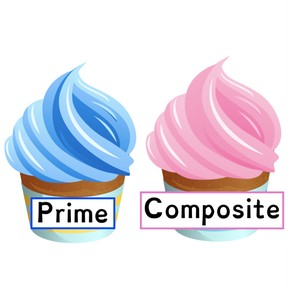Prime and composite numbers up to 100

# Prime and composite numbers up to 100

I can determine whether a number is prime or composite.8,000 schools use Gynzy92,000 teachers use Gynzy1,600,000 students use Gynzy

## General

In this lesson, students learn and practice using a variety of strategies to determine which numbers are prime and which are composite.

## Standards

CCSS.MATH.CONTENT.4.OA.B.4

## Learning objective

Students will be able to determine whether a given whole number in the range 1-100 is prime or composite.

## Introduction

Start by reviewing different fact families and have students write the missing numbers. Go over multiplication terms like factor and product. Students drag the image to demonstrate the array of the given multiplication problem.

## Instruction

Explain that composite numbers have multiple factors. Students then drag the numbers onto the lines to make facts that equal 12. Explain that these multiple factors show that 12 is a composite number. After this, go over prime numbers like the number 3 which has exactly two factors, the number 1 and itself. Take a moment to explain that number one is a special exception and its only factor is itself. Review some common rules for division and show how these can help you determine whether a number is prime or composite. Count multiples of 2 and share that even numbers are always composite. However, not all odd numbers are prime.

Students then drag numbers to the correct category (prime or composite). They also determine the multiples of the number 35. Go over a checklist to determine whether numbers are prime or composite. This includes checking if the number is even, if it adheres to the 3 or 5 rules, and possibly using a calculator. Students then drag the numbers to the category showing the strategy used to help find the answer.

## Quiz

Students respond to 10 multiple-choice and true or false questions.

## Closing

There are two cupcakes on the board labeled ‘prime’ and ‘composite.’ Students drag the birthday candle that shows their birth date onto the corresponding cupcake.

### The online teaching platform for interactive whiteboards and displays in schools

• Save time building lessons

• Manage the classroom more efficiently

• Increase student engagement